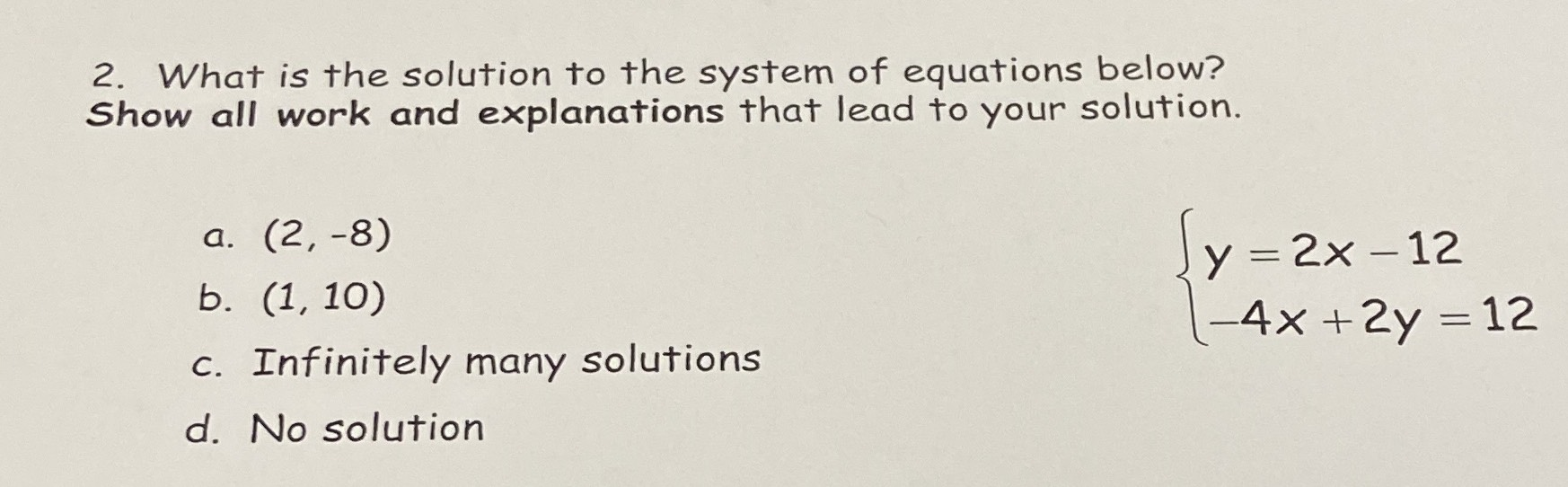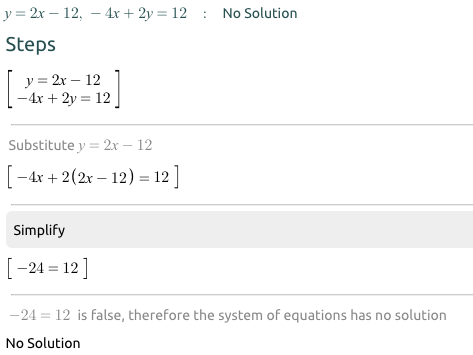### ¿Todavía tienes preguntas de matemáticas?

Pregunte a nuestros tutores expertos
Algebra
Pregunta2. What is the solution to the system of equations below? Show all work and explanations that lead to your solution.

$$\left\{ \begin{array} { l } { y = 2 x - 12 } \\ { - 4 x + 2 y = 12 } \end{array} \right.$$

a. $$( 2 , - 8 )$$

b. $$( 1,10 )$$

c. Infinitely many solutions

d. No solution